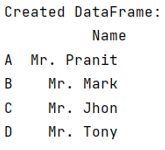# Appending pandas DataFrames generated in a for loop

Learn, how to append pandas DataFrames generated in a for loop?
Submitted by Pranit Sharma, on July 01, 2022

## Problem statement

Suppose, we are using a list of elements and iterating over them and we want to create a DataFrame by performing some operations on these elements and want to append these values in a DataFrame. If we append each value directly inside the loop, it will overwrite the previous value and only the last values will be added to the DataFrame.

## Appending pandas DataFrames generated in a for loop

To append pandas DataFrame generated in a for a loop, we will first create an empty list and then inside the loop, we will append the modified value inside this empty list, and finally, outside the loop, we will concat all the values of the new list to create DataFrame.

Note

To work with pandas, we need to import pandas package first, below is the syntax:

```import pandas as pd
```

Let us understand with the help of an example,

## Python program for appending pandas DataFrames generated in a for loop

```# Importing pandas package
import pandas as pd

# Creating a List of some values
list = ['Pranit','Mark','Jhon','Tony']

# Defining an empty list
list_2 = []

# Generating new values inside a for loop
for value in list:
dataframe_values = 'Mr. '+value

# Appending this new value in new list
list_2.append(dataframe_values)

# Finally concatenating all the values to
# create DataFrame
df = pd.DataFrame(list_2, columns=['Name'],index=['A','B','C','D'])

# Display DataFrame
print("Created DataFrame:\n",df,"\n")
```

## Output